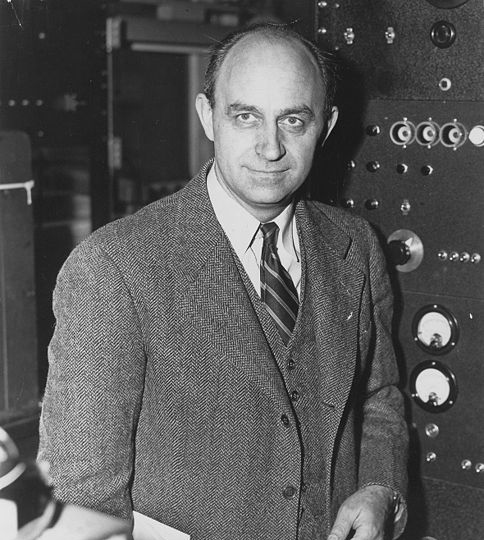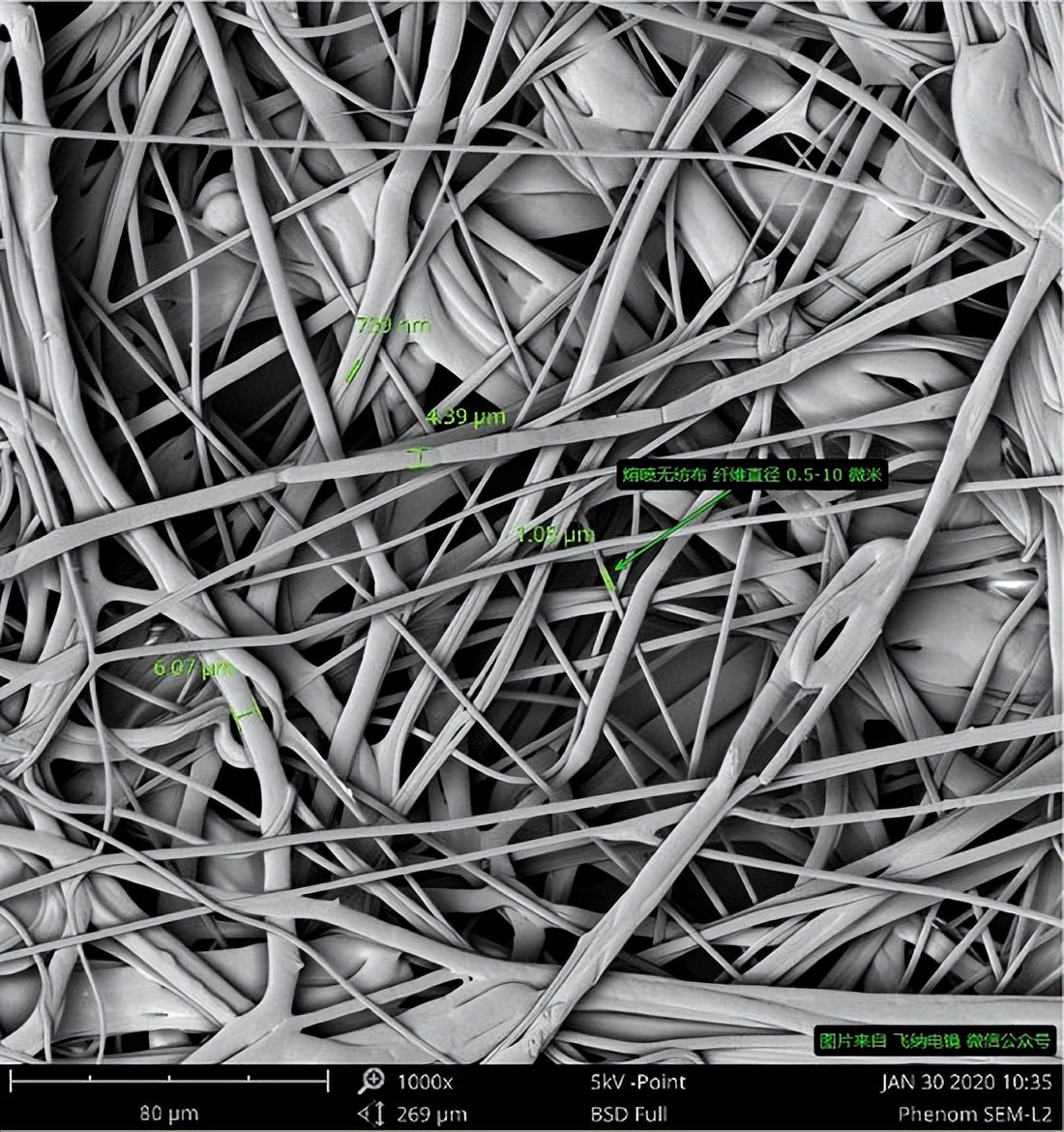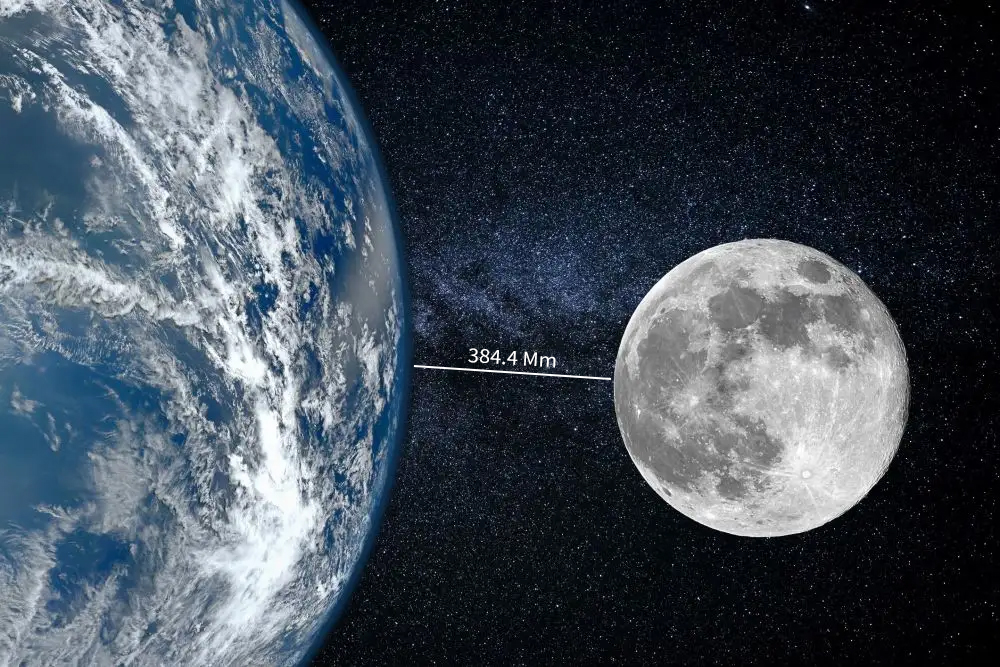# hm是什么(大小长度物理单位简介)1尧米=1000泽米=10^21千米=10^24米≈10^8光年（1Ym=1000Zm）

1泽米=1000艾米= 10^18千米=10^21米≈100000光年（1Zm=1000Em）

1艾米=1000拍米=10^15千米=10^18米≈105.7光年（1Em=1000Pm）

1拍米=1000太米=10^12千米=10^15米=一万亿千米（1Pm=1000Tm）

1太米=1000京米=10^9千米=10^12米（1Tm=1000Gm）

1京米=1000兆米=10^6千米=10^9米（1Gm=1000Mm）

1兆米=1000千米=10^6米（1Mm=1000km）

1千米=1000米=10^3米（1km=1000m）

1百米=100米=10^2米（1hm=100m）

1十米=10米（1dam=10m）

1米=10分米（1m=10dm）

1分米=10厘米=0.1米（1dm=10cm）

1厘米=10毫米=10^-2米（1cm=10mm）

1毫米=1000微米=10^-3米=0.001米（1mm=1000μm）

（废除）1毫米=10丝米（1mm=10dmm）

（废除）1丝米=10忽米（1dmm=10cmm）

（不常用）1忽米=10微米（1cmm=10μm）

1微米=1000纳米=10^-6米（1μm=1000nm）

1纳米=1000皮米=10^-9米（1nm=1000pm）

1皮米=1000飞米=10^-12米（1pm=1000fm）

1飞米=1000阿米=10^-15米（1fm=1000am）

1阿米=1000仄米=10^-18米（1am=1000zm）

1仄米=1000幺米=10^-21米（1zm=1000ym）

1幺米= 10^-24米（目前最小长度单位）

1、幺米（幺，攸米，皆米，ym，yoctometer）

2、仄米（仄，介米，zm，zepto）

3、阿米（阿，渺米，am，attometer）

4、飞米（飞，费米，fm，femtometer）1938年诺贝尔物理学奖得主。

1942年，费米领导小组在芝加哥大学建立了人类第一台可控核反应堆、为第一颗原子弹的成功爆炸奠定基础，人类从此迈入原子能时代，而费米也被誉为“原子能之父”。

5、皮米（皮，微微米，pm，picometer）

6、纳米（纳，奈米，毫纳米，nm，Nanometer）7、微米（微，μm，micron）emmm，一般头发丝大概就60~90μm，可以拔一根看看。

8、忽米（ cmm，centimillimeter）

9、丝米（dmm，decimillimeter，已废除）

1984年，中华人民共和国国务院发布《关于在我国统一实行法定计量单位的命令》中，决定我国采用国际单位，原定计量单位词头的“丝”被废除。从此“丝”这个具有千年历史的计量单位退出了历史舞台。

10、毫米、厘米、分米、米、千米，大家都挺常用，就不用介绍了。

11、十米、百米

12、兆米（兆，马咖米，Mm，符号不可小写，与毫米区分，Megameter，）13、京米（吉，吉米，Gm，Gigametre）

14、太米（太，垓米，Tm，Terameter）

“天文单位”也是一个长度单位，符号AU，指的是地球到太阳的平均距离。15、拍米（拍，拍它，Pm，peta）

1Pm=1000000000000m=一万亿千米≈1/9.5光年

16、艾米（艾，Em）

17、泽米（泽，Zm，Zettameter）

1泽米=10^18千米=10^21米≈100000光年

18、尧米（尧，佑米，Ym，Yottameter）

1尧米=1000泽米=10^21千米=10^24米≈10^8光年